Achieve Innovative Process Improvement＋Standardization by IT System with MS 365.# Learning Multiple Regression Analysis with an SEO Example【Regression Analysis Series 3】\ この記事を共有 /
Learning Multiple Regression Analysi...

This article explains Multiple Regression Analysis using my SEO activities as example and a Dummy Variable for Qualitative data and removing Collinearity.

(Duration: 8:28)

## What are Multiple Regression Analysis and the difference from Simple Regression Analysis?

Hi, this is Mike Negami, Lean Sigma Black Belt.

This episode is the third video of the ‘Regression Analysis Series’ which is very useful in business and this is about Multiple Regression Analysis.

The previous two videos are the basics and will be the foundation for today’s content, so please watch them from the link below if you haven’t done so.

This will be a short review from the previous video. Regression Analysis is finding a Regression Equation to calculate the Objective Variable Y.

In the equation, the X’s are called Explanatory Variables. When there is only one Explanatory Variable, it’s a Simple Regression Analysis. When there are multiple, it’s a Multiple Regression Analysis.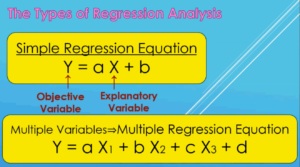In practice, we perform a Regression Analysis for sales forecasting or marketing analysis etc., but it’s rare to have only one Explanatory Variable, so we usually use Multiple Regression Analysis.

Fortunately, its operation in Excel is the same as the Simple Regression Analysis. However, when preparing source data, Multiple Regression Analysis has a couple of things you should be aware of.

## Example: Think of SEO with Multiple Regression Analysis.

I’ll also explain the Multiple Regression Analysis using this site econoshift.com as an example. These days, website management requires certain tactics to increase traffic from Google.

Those tactics are called SEO (Search Engine Optimization). In SEO, it’s essential to set Target Keywords for each page of a web site, so I always have trouble choosing the best one.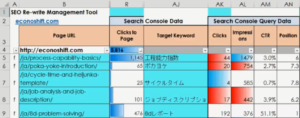The image above is a snip of the SEO tool I made. The numbers in Column R are the ‘Clicks to Page’ that came from Google to each page of my blog site. If you click on ‘Target Keywords’ in Column AJ, you can see actual search keywords that were used when they came to the page. (See the image below.)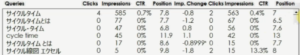There are also various data for the keywords. I would like to know which of these data are the most important for selecting Target Keywords using a Multiple Regression Analysis.

## Procedure for Multiple Regression Analysis in Practice

### Decide the Objective Variable and Explanatory Variables from your source data.

In Multiple Regression Analysis, the most important and difficult thing is to decide the Objective Variable and the Explanatory Variables effectively.

In our example, our goal is to gain more traffic from Google, so I chose the ‘Clicks to Page’ as an Objective Variable. The Explanatory Variables are selected Target Keywords’ ‘Clicks’, ‘Impressions’, ‘CTR’ and ‘Position’.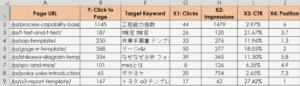Although I will not explain the details of SEO today, among those Keywords’ data, I’ll find which one influences the ‘Clicks to Page’ most with a Multiple Regression Analysis.

In our case, the data to be set as the Objective Variable was obvious, but there are cases when there are several choices. If so, it will be easier to decide by considering what you want to accomplish from the analysis results.

There are also times when you have many data to use as Explanatory Variables. However, if you include too many of them, the accuracy of the analysis will fall, so generally speaking, have up to seven Explanatory Variables at most. When you select Explanatory Variables, there are a couple of things to consider.

### What you can do with Qualitative data that is not Quantitative? ⇒ Use Dummy Variables.

Continuous data such as length and time are called Quantitative Data. On the other hand, data such as ‘Male / Female’ and ‘Like / Dislike’ are called Qualitative Data. These data are always included in survey results.

You can use those data in Multiple Regression Analysis too, but they need to be quantified. You can apply numbers like 1 for male, 2 for female, or 3 for Like, 2 for Neutral, 1 for Dislike and so on. This variable is called a Dummy Variable.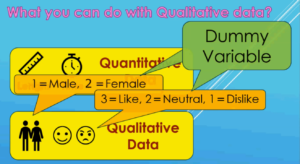### Exclude Multicollinearity. Utilize the CORREL Function.

Here is another consideration. During Multiple Regression Analysis, if you use data with high correlation among Explanatory Variables, we know that mathematically, it’s not possible to obtain a correct Regression Equation. We say that there is Multicollinearity.

In a frequently used example, when you do Multiple Regression Analysis with people’s heights as the Objective Variable and the lengths of right and left legs as Explanatory Variables, you’ll get a funny result like this: the left legs’ coefficient is minus, which means that the longer it becomes, the lower the height becomes.To prevent multicollinearity, first, calculate all of the Coefficient of Correlations between all Explanatory Variables. If there is a group of data with strong correlation, exclude one of them. Or, for some reason, if you need to include both, merge them as one group of data in an appropriate way such as averaging them.

Let’s do it on Excel. First make a cross table of all Explanatory Variable data as shown in the image below. Use the CORREL Function in each cell intersected among the variables by selecting two those data ranges to compare. It will show the Coefficient of Correlation between each two data groups.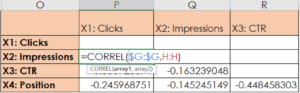I confirmed that there are no high Coefficient of Correlations this time, so I’ll use all of the data as Explanatory Objectives.

### Perform Multiple Regression Analysis with Excel Data Analysis Tool.

Let’s do a Multiple Regression Analysis now. Select ‘Data’, ‘Data Analysis’, then ‘Regression’. In the ‘Input Y Range’, select the range of the Objective Variable, which is ‘Clicks to Page’. In the ‘Input X Range’, select the entire ‘Explanatory Variables’ data range.For a Multiple Regression Analysis, you should include each column name and check ‘Labels’ (See the red arrow in the image above). As a result, the column names of your Explanatory Variables will appear in the regression result.

I explained the P-Value in the previous video in detail. Please watch it. ⇒”Simple Regression Analysis Interpretation (Excel Data Analysis Tools)【Regression Analysis Series 2】”

Briefly, Explanatory Variables with lower P-Values have stronger relationships with the Objective Variable.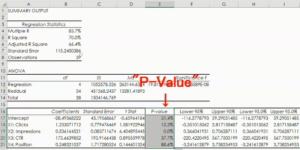In our case, it turned out that statistically the ‘Impression’ of search keywords affects the ‘Clicks to Page’ most. It was great to explain this logically.

From these coefficients, my Multiple Regression Equation looks like this:

Y = 1.23 ‘Clicks’ + 0.54 ‘Impression’ + 173.66 ‘CTR’ + 0.25 ‘Position’ – 28.49

Now I can predict the ‘Clicks to Page’ from my Target Keywords data. The ‘Adjusted R Square’ is 66% so it is a reasonable result.

The best result in this analysis for me was to find that ‘Impression’ is the most important factor when deciding my Target Keywords. This is very helpful for my SEO activities.

“See these other popular articles.”

### 最新記事